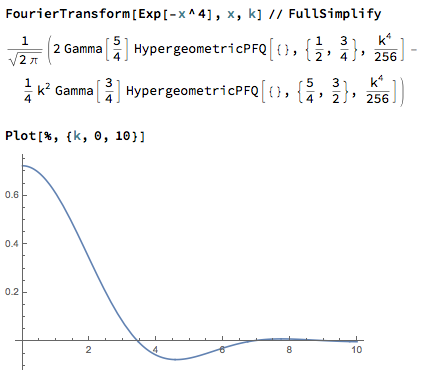# Positivity of certain Fourier transform

Is the Fourier transform of the function $$f(\xi) = e^{-t|\xi|^{2m}}$$ positive for $t>0$ and $m \in \mathbb{N}_0$?

• I love it when simple questions like this one generate such wonderful answers – almaz Dec 6 '16 at 22:45

it is positive for $$m=1$$, but not for $$m=2$$, see this Mathematica output:• Sorry, I should have done this myself. Thought too abstractly about it -.- – Matthias Ludewig Dec 6 '16 at 10:38

A simple argument that the Fourier transform cannot be nonnegative for any $m>1$, integer or not, is given in my 1991 paper with Odlyzko and Rush:

Noam D. Elkies, Andrew M. Odlyzko, and Jason A. Rush: On the packing densities of superballs and other bodies, Invent. Math. 105 (1991), 613-639.

The Fourier transform $f$ of a nonnegative even function must satisfy $4f(\xi) \leq 3 f(0) + f(2\xi)$ for all $\xi$. This fails for any $m>1$ once $\xi$ is close enough to (but not equal) zero.

Postscripts:

0) The inequality $4f(\xi) \leq 3 f(0) + f(2\xi)$ holds because the difference is $\int_{-\infty}^\infty \hat f(\eta) \, w(\eta) \, d\eta$ with $w(\eta) \geq 0$ for all $\eta$ because $3 - 4\cos \theta + \cos 2\theta = 8 \sin^4 (\theta/2) \geq 0$.

1) The nonnegativity of $3 - 4\cos \theta + \cos 2\theta$ is also a key ingredient in the proof of thenonvanishing of the Riemann zeta function $\zeta(s)$ on the edge $s = 1+it$ of the critical strip, and thus of the Prime Number Theorem. See the last exercise of this chapter my notes on analytic number theory.

2) Sergei noted that it is also known that for $0 < m \leq 1$ the Fourier transform is positive. As I wrote earlier this year on Math Stackexchange, the same paper with Odlyzko and Rush also proves that fact as follows, crediting the argument to B.F. Logan (see Lemma 5 on page 626).
We first prove this for $m=1$, then reduce $0<m<1$ to this case. For $m=1$, the function $\exp(-t|\xi|^{2m})$ is a Gaussian, and thus so is its Fourier transform; Gaussians are positive everywhere, so the case $m=1$ is done. For $0<m<1$, the function $\exp(-t|\xi|^{2m})$ is a nonnegative mixture of Gaussians $\exp(-c\xi^2)$ by Bernstein's theorem. Therefore the Fourier transform is also a nonnegative mixture of Gaussians, so again positive everywhere. QED

I think the result goes back to Polya, see "Some theorems on stable processes" by Blumenthal and Getoor. Another reference is Paul Lévy "Sur une application de la dérivée d’ordre non entier au calcul des probabilités" page 1118 of CRAS 1923.

It might be good to also give the gist of Levy's very simple argument: if the Fourier transform was positive then the exponent would be the log-moment generating function of a random variable $X$. But if $m>1$, the second derivative at the origin vanishes and thus also the variance of $X$. The positive function would be a delta function which is a contradiction.

• Due to information in 2013 V.P.Zastavny thesis a problem was first in more general form formulated in Schoenberg,1938,Tran.AMS 44. Interesting generalizations are in Zastavnyi V. P. Positive definite functions depending on the norm / V. P. Zastavnyi // Russian J. Math. Physics. – 1993. – Vol. 1,ð4. – P. 511– 522. and Koldobskii : mathnet.ru/php/… – Sergei Dec 7 '16 at 6:12
• @Sergei: thanks for pointing out the work of Zastavnyi. However, the latter seems to consider the (more difficult) case where the norm involved is $L^p$ with $p>2$ on $\mathbb{R}^n$, $n\ge 2$. – Abdelmalek Abdesselam Dec 7 '16 at 20:07

This is a well-known problem, solved recently in dissertation of Zastavny based on some inequalities for p.d.f. More: it is a Schonberg problem, positive for $0<m\leq1$, not positive otherwise.